Courses

# Evaporation Loss Of Water In Cooling Tower Chemical Engineering Notes | EduRev

## Chemical Engineering : Evaporation Loss Of Water In Cooling Tower Chemical Engineering Notes | EduRev

The document Evaporation Loss Of Water In Cooling Tower Chemical Engineering Notes | EduRev is a part of the Chemical Engineering Course Mass Transfer.
All you need of Chemical Engineering at this link: Chemical Engineering

6.7 Evaporation loss of water in cooling tower:

Blowdown: During the cooling process of hot water in cooling tower, around 2% water evaporates [1-3]. In the long run, it increases the solid content in the circulating water. Some dust particles also come from the environment and mix with circulating water. But the solid content of the cooled water must be kept under a certain limit to avoid scaling or fouling on the heat exchange equipment. A part of the circulating water is drained from the bottom of the cooling tower to discard the deposited solids from the cooling tower. This is called blowdown. The losses due to blowdown, evaporation, drift and leakage are compensated by adding make-up water.

Water balance in cooling tower
M=B+D+E                                                   (6.38)
where, M is make-up water rate; B is blowdown rate; D is drift leakage loss rate; E is evaporation loss.
Solid balance
M×C1=(B+D) ×C2+E×0                                      (6.39)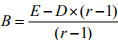(6.40)
where, r=C2/C1; C1 is dissolved solid concentration in the make-up water; C2 is dissolved solid concentration in the circulating water.

Evaporation loss is estimated by a thumb rule as:
E=water flow rate (L)×range(ºF)×0.00085 gallon/min

The other design characteristics are pump horsepower, fan horsepower, source of make-up water and drift eliminators.

Nomenclature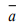Contact area/tower volume, m2/m3
L           Water flow rate, kg/m2s
cwL       Heat capacity of liquid (water), kJ/kg.K
V           active cooling volume/plan area, m3/m2
Gs          Air rate, kg dry air/m2s
Y/           Humidity, kg moisture/kg dry air
hG           Heat transfer coefficient of air film, kJ/m2.s.K
Y/w        Saturation humidity, kg moisture/kg dry air
hL          Heat transfer coefficient of liquid (water), kJ/m2.s.K
z          Cooling tower height, m
kG          Mass transfer co-efficient of moisture transport, kg/m2.s (ΔpA)
λw          Latent heat of vaporization of water, kJ/kg
K/Y          Overall mass transfer co-efficient, kg/m3.s
VH          Humid volume, m3/kg dry air

HUMIDIFICATION PROBLEM
Example Problem 6.1:
A cooling tower is to be designed to cool water from 450C to 300C by countercurrent contact with air of dry bulb temperature 300C and wet bulb temperature of 250C. The water rate is 5500 kg/m2.h and the air rate is 1.25 times the minimum. Determine the tower height if the individual gas-phase mass transfer coefficient (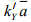) is 5743.5
kg/m3 h (ΔY). The volumetric water side heat transfer coefficient is given by hLā=0.059L0.51Gs, in Kcal/m3hK, where L and Gs are mass flow rates of water and air (dry basis). Antoine Equation: ln PVA (bar)=11.96481-3984.923/(T-39.724).

Solution 6.1:
TG1=30ºC
Tw=Tas=25ºC
Y/1 =0.019 [From psychrometric chart]
H/1= {(1.005 1.88 0.019) 30 2500 0.019}kJ/kg
=78.7 kJ/kg
TL1=30ºC
Locate point Q(TL1, H/1 ) (Lower terminal of operating line) at Q(30, 78.7) on TL-Hplane.

Generation of Equilibrium curve
(i) Calculate pv from Antoine Equation: ln PVA (bar)=11.96481-3984.923/(T39.724).
(ii)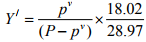(iii)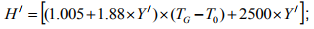Here, reference temperature, T0 is 0ºC.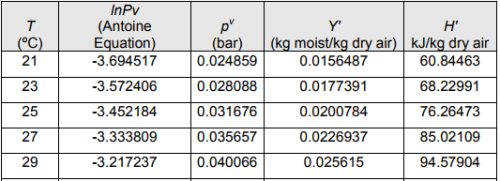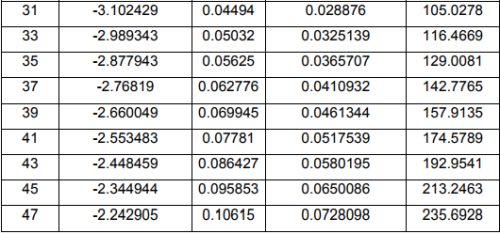Draw a tangent to the equilibrium line through Q. Slope of the tangent is 8.78.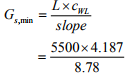=2623 kg/h.m2
Actual air rate =Gs,min×1.25
=2623×1.25 kg/h.m2
= 3279 kg/h.m2
Slope of the operating line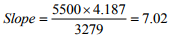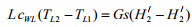5500 x 4.187(45 - 30) = 3279(H/2 - 78.7)
H/2 = 184kJ/kg
Now, locate point P (TL2, H/2 ) (Upper terminal of the operating line) at P (45, 184) on TL-Hplane.

We have,
hLā=0.059L0.51Gs
=0.059(5500)0.51(3279) kcal/m3h.K
=15637.9 kcal/m3hK=15637.9 ×4.187 kJ/m3h.K
=65,475.9 kJ/m3hK
Slope of tie line=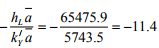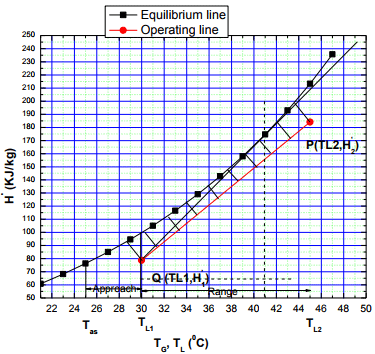A set of tie lines of this slope is drawn from several points on the operating line. These tie lines meet the equilibrium line at (TLi, H/i ). Hence, the points (H/ , H/i ) are obtained. The values of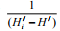are plotted against TL and the integral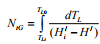is evaluated graphically.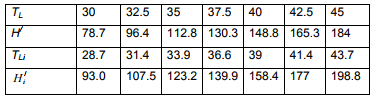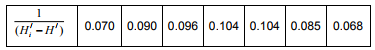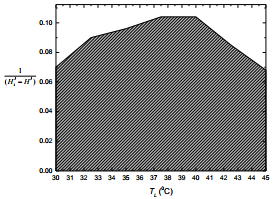NtG=Area under the curve= (184-78.7)×0.088=9.27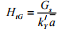=(3279/5743.5)m=0.56 m
Tower height= 9.27×0.56 m=5.19 m (Ans.)

Offer running on EduRev: Apply code STAYHOME200 to get INR 200 off on our premium plan EduRev Infinity!

,

,

,

,

,

,

,

,

,

,

,

,

,

,

,

,

,

,

,

,

,

;